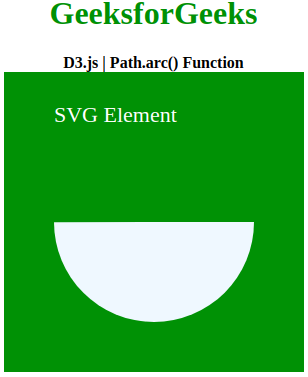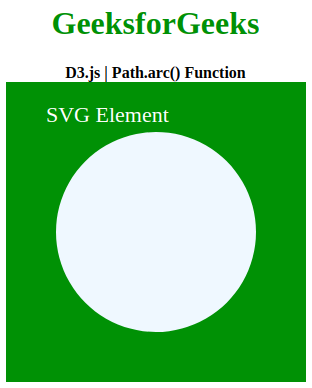Related Articles

# D3.js | Path.arc() Function

• Last Updated : 22 Jun, 2020

D3.js is mostly used for making of graph and visualizing data on the HTML SVG elements. D3.js has many functions one of which is arc() function. The Path.arc() function is used to make a arc and a circle and other shapes. D3 stands for Data Driven Documents and mostly used for data visualization.

Syntax:

Hey geek! The constant emerging technologies in the world of web development always keeps the excitement for this subject through the roof. But before you tackle the big projects, we suggest you start by learning the basics. Kickstart your web development journey by learning JS concepts with our JavaScript Course. Now at it's lowest price ever!

```path.arc(x, y, radius, startAngle, endAngle[anticlockwise])
```

Parameters: This function accepts five parameter as mentioned above and described below:

• x: This parameter holds the x-position of the arc in the SVG.
• y: This parameter holds the y-position of the arc in the SVG.
• startAngle: This parameter holds the start angle of the arc.
• endAngle[anticlockwise]: This parameter holds the  angle to which the arc is to be made,  if anticlockwise is true, the arc is drawn in the anticlockwise direction.

Note: If the current point is not equal to the starting point of the arc, a straight line is drawn from the current point to the start of the arc.

Below example illustrate the D3.js | Path.arc() Function in D3.js:

Example 1:

## HTML

 ```<``html` `lang``=``"en"``>``   ``<``head``>``      ``<``meta` `charset``=``"UTF-8"``>``      ``<``meta` `name``=``"viewport"` `            ``path1tent="``width``=``device``-width, ``                       ``initial-scale``=``1``.0">``      ``<``title``>D3.js| Path.arc() Function``   ````   ``<``style``>``      ``body {``          ``text-align: center;``      ``}``      ``h1 {``          ``color: green;``      ``}``      ``svg{``      ``background-color: green;``      ``}``      ``.path1{``      ``fill: aliceblue;``      ``}``   ````   ``<``body``>``      ``<``h1``>GeeksforGeeks``      ``<``b``>D3.js | Path.arc() Function``      ``<``div``> ``         ``<``svg` `width``=``"300"` `height``=``"300"``>``            ``<``text` `x``=``"50"` `y``=``"50"` `font-family``=``"Verdana"` `                  ``font-size``=``"22"` `fill``=``"white"``>``                ``SVG Element``            ````            ``<``path` `class``=``"path1"``>``         ````      ````      ``<``script` `src` `= ``"https://d3js.org/d3.v4.min.js"``>``      ````      ``<``script``>``         ``// Creating path object``         ``var path1= d3.path();``          ` `         ``// Creating arc of radius 100``         ``path1.arc(150,150,100,0,3.14)``         ``d3.select(".path1").attr("d",path1);``      ````   `````

Output:Example 2:

## HTML

 ```<``html` `lang``=``"en"``>``   ``<``head``>``      ``<``meta` `charset``=``"UTF-8"``>``      ``<``meta` `name``=``"viewport"` `            ``path1tent="``width``=``device``-width, ``                       ``initial-scale``=``1``.0">``      ``<``title``>D3.js| Path.arc() Function``   ````   ``<``style``>``      ``body {``          ``text-align: center;``      ``}``      ``h1 {``          ``color: green;``      ``}``      ``svg{``      ``background-color: green;``      ``}``      ``.path1{``      ``fill: aliceblue;``      ``}``   ````   ``<``body``>``      ``<``h1``>GeeksforGeeks``      ``<``b``>D3.js | Path.arc() Function``      ``<``div``> ``         ``<``svg` `width``=``"300"` `height``=``"300"``>``            ``<``text` `x``=``"40"` `y``=``"40"` `font-family``=``"Verdana"` `                  ``font-size``=``"22"` `fill``=``"white"``>``                ``SVG Element``            ````            ``<``path` `class``=``"path1"``>``         ````      ````      ``<``script` `src` `= ``"https://d3js.org/d3.v4.min.js"``>``      ````      ``<``script``>``         ``// Creating path object``         ``var path1= d3.path();``          ` `         ``// Creating arc of radius 100``         ``path1.arc(150,150,100,0,2*3.14)``         ``d3.select(".path1").attr("d",path1);``      ````   `````

Output:My Personal Notes arrow_drop_up